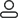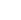LanguageLogin|Sign up
Blog >> Blog Details Page
The influence and calculation of the PCB trace
Posted:02:37 PM April 25, 2018 writer: G

The influence of the right-angle trace on the signal is mainly reflected in three aspects:

First, the corner can be equivalent to the capacitive load on the transmission line, slowing the rise time;

secondly, the impedance discontinuity will cause reflection of the signal;

and third, the right-angle tip will be generated. EMI.

The parasitic capacitance due to the right angle of the transmission line can be calculated by the following empirical formula:

C=61W(Er)1/2/Z0

In the above equation, C refers to the equivalent capacitance of the corner (unit: pF), W refers to the width of the trace (unit: inch), εr refers to the dielectric constant of the dielectric, and Z0 is the characteristic impedance of the transmission line. For example, for a 4-Mils 50-ohm transmission line (εr of 4.3), a right-angle brings about 0.0101pF of capacitance, which can be used to estimate the amount of rise time variation caused by this:

T10-90%=2.2*C*Z0/2 = 2.2*0.0101*50/2 = 0.556ps

It can be seen from the calculation that the capacitive effect brought by the right-angle trace is extremely small.

As the line width of the right angle trace increases, the impedance at this point will decrease, and a certain signal reflection phenomenon will occur. We can calculate the equivalent impedance after the line width is increased according to the impedance calculation formula mentioned in the transmission line section, and then The reflection coefficient is calculated according to an empirical formula: ρ=(Zs-Z0)/(Zs+Z0). Generally, the impedance change caused by a right-angled trace is between 7% and 20%, and thus the maximum reflection coefficient is about 0.1. Moreover, as can be seen from the figure below, the impedance of the transmission line changes to the minimum during the W/2 line time, and then returns to the normal impedance after W/2 time, the entire impedance change time is very short, often at 10ps. Internally, such rapid and minor changes are almost negligible for general signal transmission.

Many people have such an understanding of right-angle alignment that the tip is easy to emit or receive electromagnetic waves, resulting in EMI, which has become one of the reasons why many people think that it is not possible to line at right angles. However, the results of many practical tests show that right-angle traces do not produce significant EMI compared to straight lines. Perhaps the current instrument performance and test level limit the accuracy of the test, but it at least illustrates a problem that the radiation of the right-angle trace is less than the measurement error of the instrument itself. .

In general, right-angle alignments are not as frightening as they are. At least in applications below GHz, any effects such as capacitance, reflection, and EMI that are produced in the TDR test can hardly be reflected. High-speed PCB design engineers should focus on layout, power/ground design, and trace design. Other aspects such as vias. Of course, although the influence of right-angle alignments is not very serious, it does not mean that we can all follow the right-angled lines. Attention to detail is an essential quality for each outstanding engineer. Moreover, with the rapid development of digital circuits, PCB The frequency of signals processed by engineers will also continue to increase, and in the area of RF design above 10 GHz, these small right angles may become the focus of high-speed problems.

885 1 0 1
• PCB
Prototype
• PCB
Assembly
• SMD
Stencil
 Dimensions: (mm) × Quantity: (pcs) 5 5 10 15 20 25 30 40 50 75 100 120 150 200 250 300 350 400 450 500 600 700 800 900 1000 1500 2000 2500 3000 3500 4000 4500 5000 5500 6000 6500 7000 7500 8000 9000 10000 Other Quantities:(quantity*length*width is greater than 10㎡) OK Layers: 1 2 4 6 8 10 12 Thickness: 0.6 mm 0.8 mm 1.0 mm 1.2 mm 1.6 mm 2.0 mm 2.5 mmQuote now